Next: The Pairwise Comparison of Up: Insertions and Deletions Previous: Insertions and Deletions

# Gap Probabilities and Penalties

Our algorithms for aligning amino acid sequences are based on the test of whether the two sequences evolved from a common ancestral sequence or not. This model allowed us to derive scores (used by the dynamic programming algorithm) from the mutation probabilities. In this section, we derive gap penalty costs from the probabilities of a gap occuring.

The gap model we use here is the one which is implicit in the vast majority of the present literature. This assumes that gaps are accidents at the DNA level which produce a random insertion or deletion of k amino acids with probability q(k). This probability is independent of the amino acids being inserted or deleted and of any neighbouring amino acids. It is a natural assumption that this probability also depends on the PAM distance t between the two sequences, in particular, gaps in similar sequences are less frequent than gaps in distant ones. ThenGap penalties used with dynamic programming are typically of the form a+bk. In some cases the values of a and b depend on the PAM distance t. This gap penalty function used for dynamic programming will be denoted by d(k,t). For example

d(k,t) = a+bk

The linear gap penalty function is very popular, in part because it is possible to compute dynamic programming alignments very efficiently using Gotoh's algorithm.

The relation between d(k,t) and q(k,t) is simply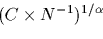This relation is not well known, so we will prove it here. Readers not interested in the mathematical theory of alignments should skip the rest of this section.

The purpose of aligning sequences with dynamic programming is to find the alignment which maximizes the probability of the two sequences having evolved from a common ancestor as opposed to being just random sequences. Let A and B be two sequences with lengths nA and nB. Let Ai be the ith amino acid in A (similarly for B). Let fi be the frequency of amino acid i as defined in the previous section. The probability that the two sequences are random isFor example, given an alignment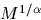where X denotes the unknown ancestral parent sequence. The probability of this alignment being the consequence of having a common ancestor is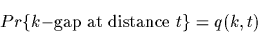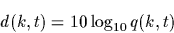for all the possible X1 which evolved into A1 on one side and into B1 on the other, and similarly for X2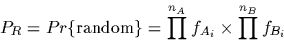where this term denotes the probability of a gap of length 3 on one sequence times the probability of the sequence B3B4B5 on the other and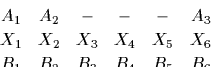for the alignment of A3 against B6. For this alignment the quotient of the probabilities is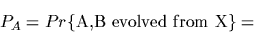once property grouped and simplified. To find the alignment which maximizes this probability we transform the maximization of a product (of all positive values) into the maximization of a sum by computing the logarithm of each term. To find the alignment which maximizes a sum, we use the dynamic programming algorithm. For historical reasons and to work with simpler numbers, these logarithms are multiplied by 10, which has no effect on the maximization process. Notice that the terms like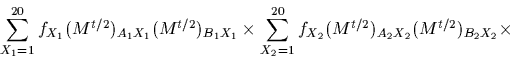are exactly the values of the standard Dayhoff matrices. The deletion cost for the above example, under dynamic programming, must be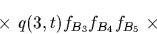It is obvious how to generalize this result for any number or length of gaps.

The relationship can be applied in two directions: either we can estimate the probabilities from a sample and compute the appropriate gap penalties or we can compute the probabilities that are implied by a gap penalty function. A linear gap penalty function implies an exponential distribution of probabilities.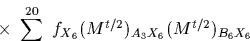implies

q(k,t) = 10a/10 (10b/10)k

For example, if a=-10 and b=-3, then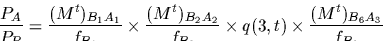This further implies that the probability of a gap, of any length, is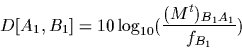in our example, the probability of having a gap would be. Finally, the expected length of a gap is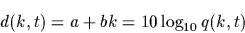in our example, the expected length of a gap would be 2.0011.Next: The Pairwise Comparison of Up: Insertions and Deletions Previous: Insertions and Deletions
Gaston Gonnet
1998-09-15